• 所有分类
• 其它

# 前言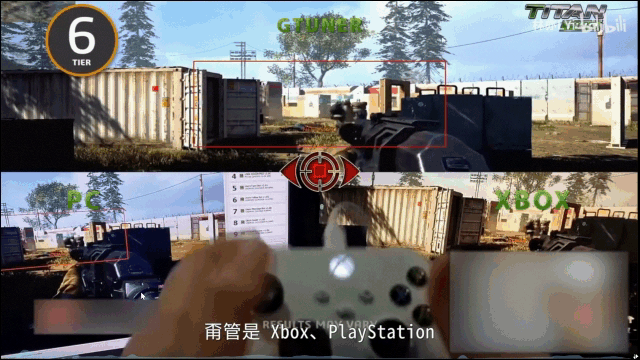# 一、核心功能设计

• 训练人体关节点检测模型
• 输入视频或图片到AI视觉模型，并输出瞄准点位置
• 自动操作鼠标移动到对应瞄准位置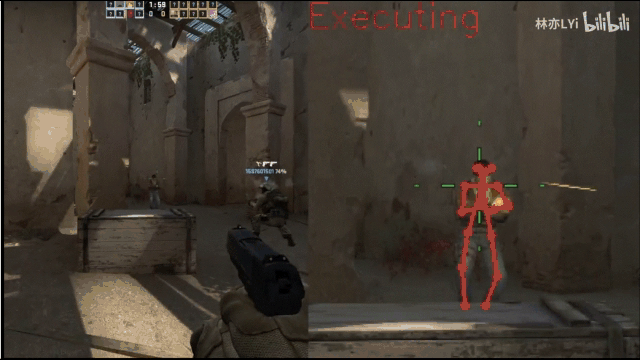# 二、核心实现步骤

## 1.训练人体关节点检测模型

### 1.1 HRNet代码库安装

``````git clone https://github.com/leoxiaobin/deep-high-resolution-net.pytorch.git
python -m pip install -e deep-high-resolution-ne.pytorch
``````

### 1.2 人体关键点数据集下载

annotations
├── captions_train2017.json
├── captions_val2017.json
├── instances_train2017.json
├── instances_val2017.json
├── person_keypoints_train2017.json    人体关键点检测对应的训练集标注文件
└── person_keypoints_val2017.json     人体关键点检测对应的验证集标注文件

datasets
├── coco
│   ├── annotations
│   ├── test2017
│   ├── train2017
│   └── val2017

### 1.3 环境配置与模型训练

``````def train(config, train_loader, model, criterion, optimizer, epoch,
output_dir, tb_log_dir, writer_dict):
batch_time = AverageMeter()
data_time = AverageMeter()
losses = AverageMeter()
acc = AverageMeter()
# switch to train mode
model.train()
end = time.time()
for i, (input, target, target_weight, meta) in enumerate(train_loader):
data_time.update(time.time() - end)
outputs = model(input)
target = target.cuda(non_blocking=True)
target_weight = target_weight.cuda(non_blocking=True)
if isinstance(outputs, list):
loss = criterion(outputs, target, target_weight)
for output in outputs[1:]:
loss += criterion(output, target, target_weight)
else:
output = outputs
loss = criterion(output, target, target_weight)
loss.backward()
optimizer.step()
# measure accuracy and record loss
losses.update(loss.item(), input.size(0))
_, avg_acc, cnt, pred = accuracy(output.detach().cpu().numpy(),
target.detach().cpu().numpy())
acc.update(avg_acc, cnt)
batch_time.update(time.time() - end)
end = time.time()
if i % config.PRINT_FREQ == 0:
msg = 'Epoch: [{0}][{1}/{2}]t'
'Time {batch_time.val:.3f}s ({batch_time.avg:.3f}s)t'
'Speed {speed:.1f} samples/st'
'Data {data_time.val:.3f}s ({data_time.avg:.3f}s)t'
'Loss {loss.val:.5f} ({loss.avg:.5f})t'
'Accuracy {acc.val:.3f} ({acc.avg:.3f})'.format(
epoch, i, len(train_loader), batch_time=batch_time,
speed=input.size(0)/batch_time.val,
data_time=data_time, loss=losses, acc=acc)
logger.info(msg)
writer = writer_dict['writer']
global_steps = writer_dict['train_global_steps']
writer_dict['train_global_steps'] = global_steps + 1
prefix = '{}_{}'.format(os.path.join(output_dir, 'train'), i)
save_debug_images(config, input, meta, target, pred*4, output,
prefix)``````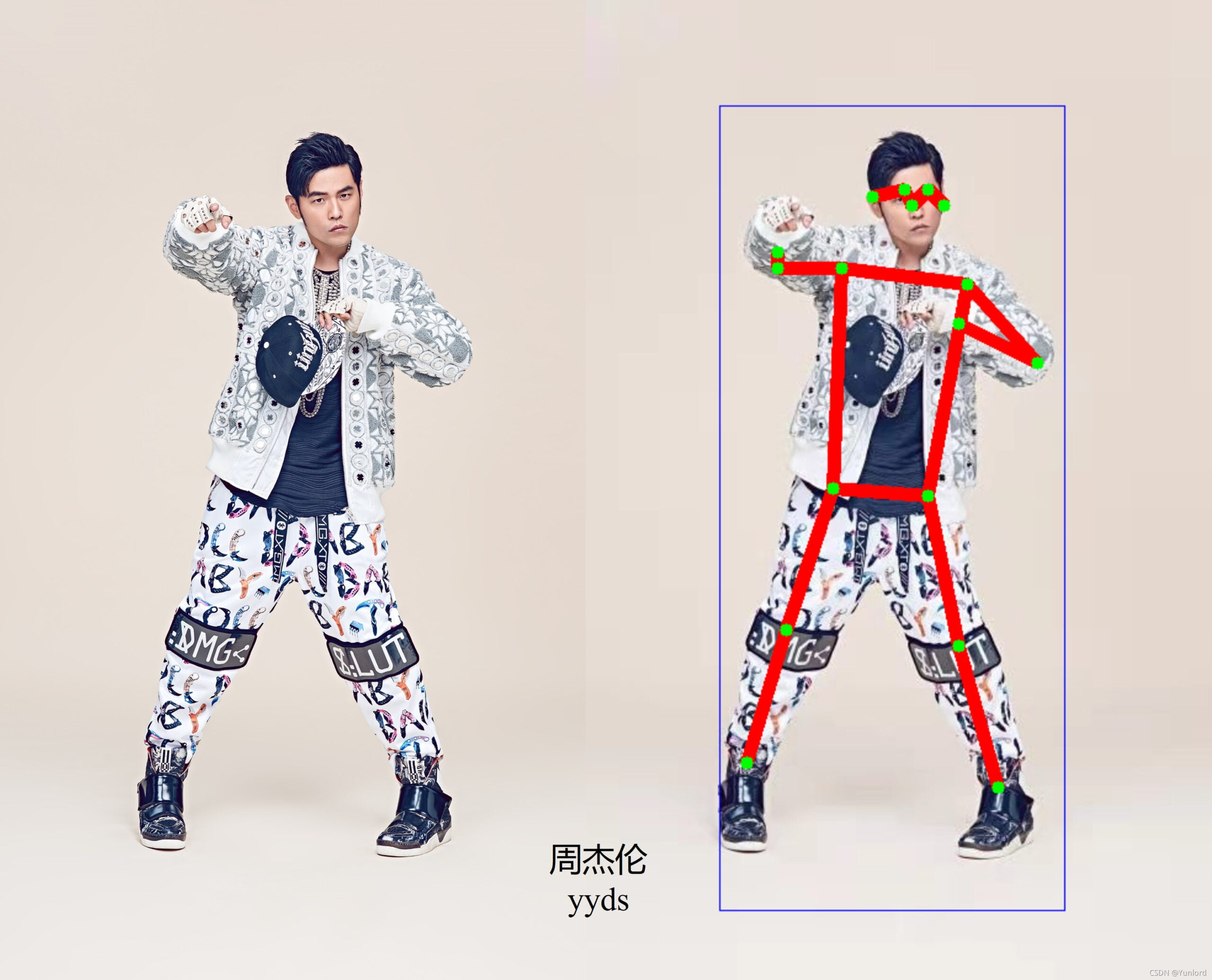## 2.输入视频或图片实时反馈瞄准点坐标

### 2.1 实时读取屏幕画面

``````import pyautogui
img = pyautogui.screenshot()
``````

``im = pyautogui.screenshot(region=(0, 0, 300 ,400))``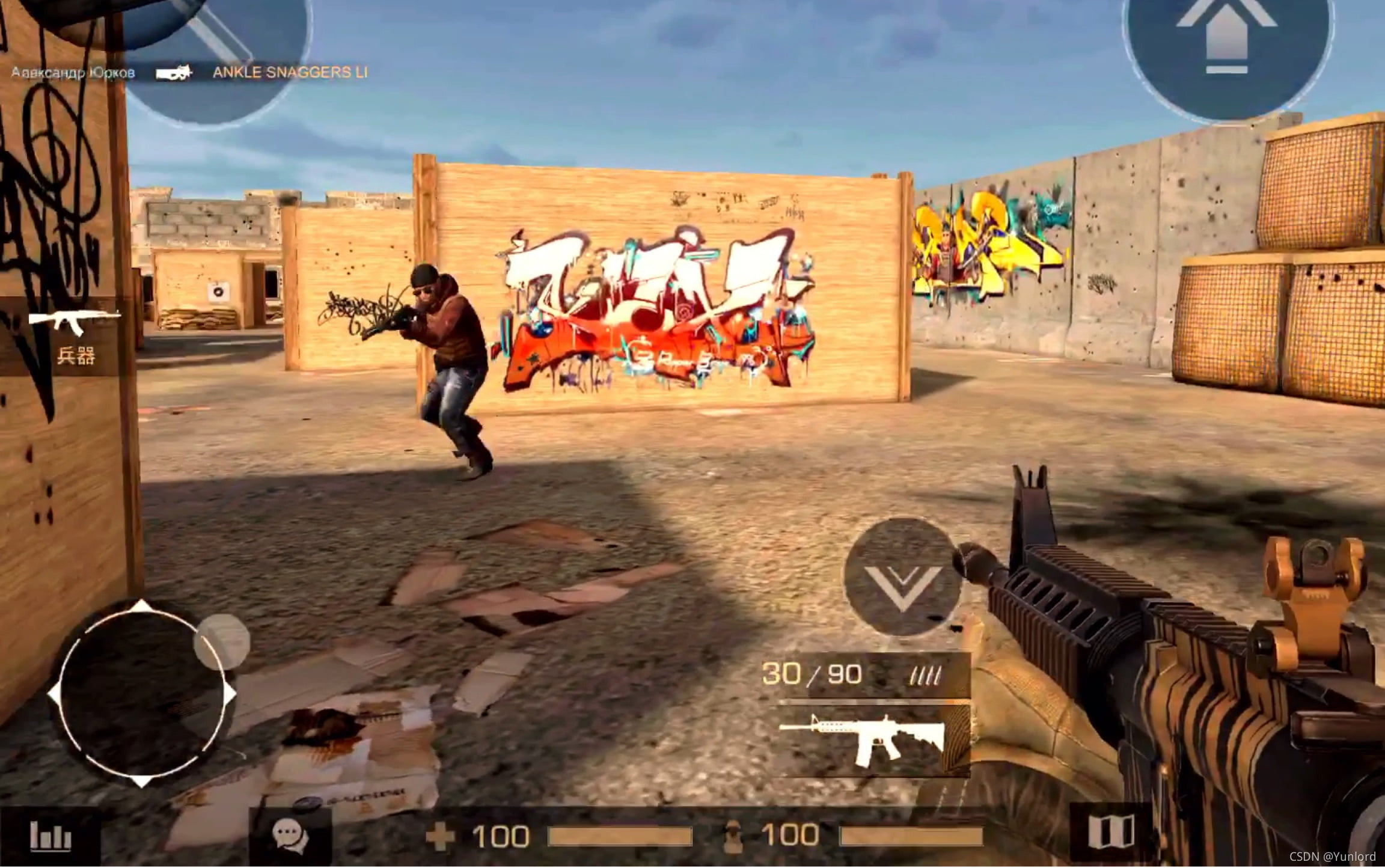### 2.2 读取图片反馈坐标

``````parser.add_argument('--keypoints', help='f:full body 17 keypoints,h:half body 11 keypoints,sh:small half body 6 keypotins')
hp = PoseEstimation(config=args.keypoints, device="cuda:0")``````

“keypoints”: { 0: “nose”, 1: “left_eye”, 2: “right_eye”, 3: “left_ear”, 4: “right_ear”, 5: “left_shoulder”, 6: “right_shoulder”, 7: “left_elbow”, 8: “right_elbow”, 9: “left_wrist”, 10: “right_wrist”, 11: “left_hip”, 12: “right_hip”, 13: “left_knee”, 14: “right_knee”, 15: “left_ankle”, 16: “right_ankle” }

``````location=hp.detect_head(img_path, detect_person=True, waitKey=0)

def detect_head(self, image_path, detect_person=True, waitKey=0):

kp_points, kp_scores, boxes = self.detect_image(bgr_image,
threshhold=self.threshhold,
detect_person=detect_person)
return kp_points``````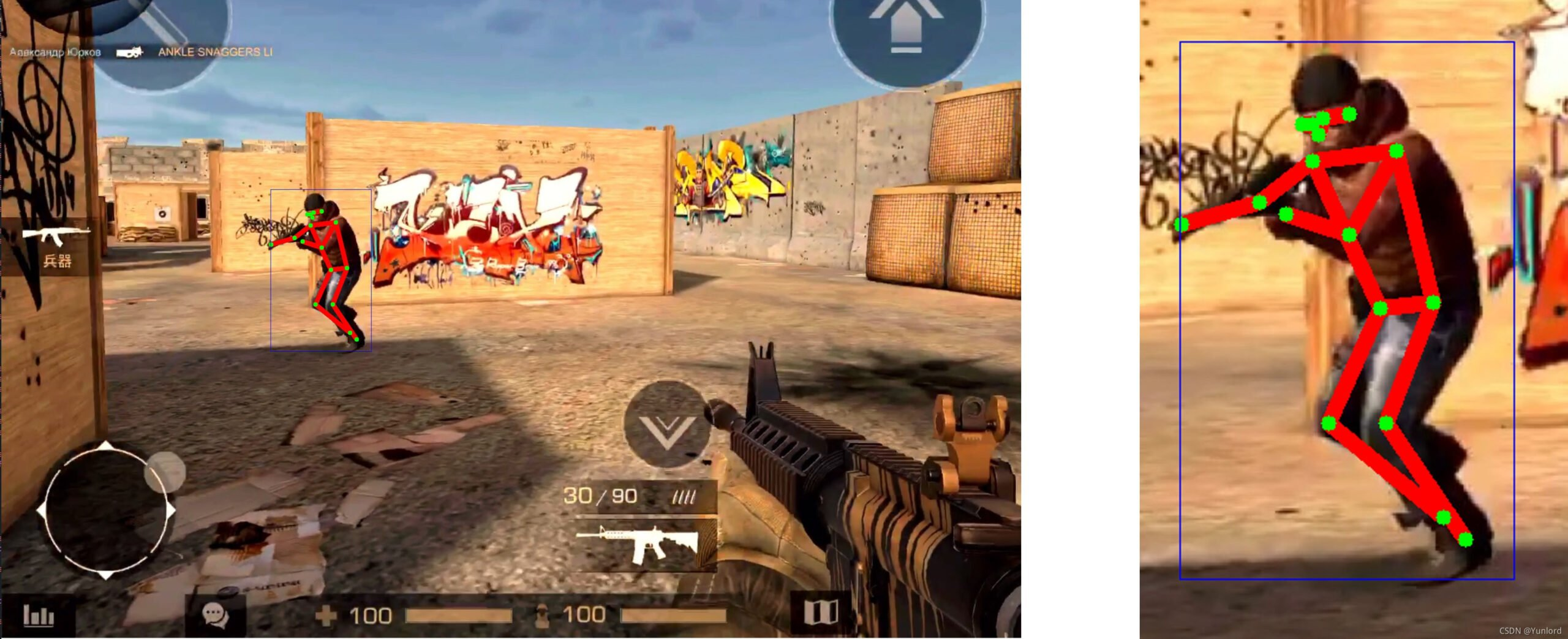## 3.自动移动鼠标到对应的坐标点

### 3.1 移动鼠标

``pyautogui.moveTo(100,300,duration=1)``

``print(pyautogui.position())   # 得到当前鼠标位置；输出：Point(x=200, y=800)``

### 3.2 控制鼠标点击

``````# 点击鼠标
pyautogui.click(10,10)   # 鼠标点击指定位置，默认左键
pyautogui.click(10,10,button='left')  # 单击左键
pyautogui.click(1000,300,button='right')  # 单击右键
pyautogui.click(1000,300,button='middle')  # 单击中间``````

``````pyautogui.doubleClick(10,10)  # 指定位置，双击左键
pyautogui.rightClick(10,10)   # 指定位置，双击右键
pyautogui.middleClick(10,10)  # 指定位置，双击中键``````

``````pyautogui.mouseDown()   # 鼠标按下
pyautogui.mouseUp()    # 鼠标释放``````

【亦】警惕AI外挂！我写了一个枪枪爆头的视觉AI，又亲手“杀死”了它

# 三、引发的思考

• 准确性
• 隐蔽性
• 通用性

up主提到可以通过算法检测游戏异常操作，这也是一种思路，但是实现起来还是比较困难，毕竟可以让AI更像人类的操作。

2D Pose人体关键点实时检测(Python/Android /C++ Demo)

PyQt5 实时获取屏幕界面图像，python3使用matplotlib